# GED Math : Statistics

## Example Questions

### Example Question #1 : Mean

Veronica went to the mall and bought several small gifts for her sister's birthday. The prices of the items were the following:

$8,$10, $6,$3, $7,$10, $2,$5, $3 What is the mean price? Possible Answers:$8

$5$6

$10$3

$6 Explanation: The mean of a set is the sum of all elements divided by the number of elements in the set: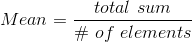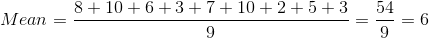The mean is$6.

### Example Question #2 : Mean

On the first two tests of the year, James scored 100 and 70, respectively. He then scored a 96 on his third test. There is just one test remaining, and James wants to finish the semester with a 90 average. What score does he need to receive on the fourth test of the semester to finish with a mean score of 90?

90

99

92

94

100

94

Explanation:

The mean in a data set of scores is the sum of all scores divided by the number of scores: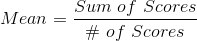Call the fourth test score. Plug in what we know and solve for: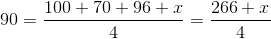Multiply both sides by 4: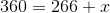Then, subtract 266 from both sides:.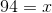To finish with a mean of 90, James must score a 94 on his fourth test.

### Example Question #7 : Mean

Calculate the mean of the following numbers: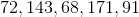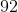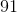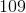Explanation: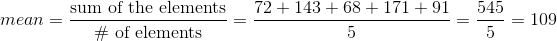### Example Question #1 : Mean

John would like to have a 90 average for math for this semester. John received a 78, 89, 100 on three of his tests. What is the lowest grade he could receive on his last test in order for him to get a 90 average for the semester?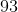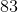Explanation:

In order for John to maintain a 90 average for the marking period, his total for four tests must be 360.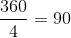or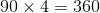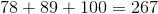If you take the total of 267, which is the total amount of points for the three tests already taken and subtract it from the 360 points which is the total amount of points needed for a 90 average, he would need to get a 93 on his last test.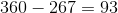### Example Question #1 : Mean

Determine the mean of the numbers: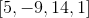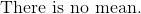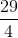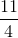Explanation:

The mean is the average of all the numbers in the set of data.

Add the numbers together and divide the sum by 4.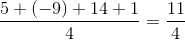The answer is:### Example Question #10 : Mean

Determine the mean of the numbers: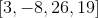Explanation:

The mean is the average of all the number ins the data set.

Add all the numbers and divide the sum by four.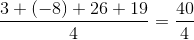The answer is:### Example Question #11 : Mean

Use the following data set of test scores to answer the question: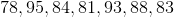Find the mean.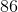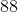Explanation:

To find the mean (or average), we will use the following formula: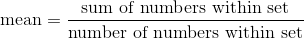So, given the setwe can calculate the following: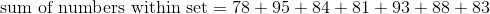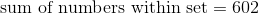We can also calculate the following: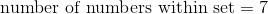because there are 7 numbers in the data set.

So, we can substitute. We get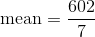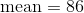Therefore, the mean of the data set is 86.

### Example Question #11 : Mean

A Science class took an exam. Here are the scores of 9 students: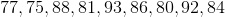Find the mean score.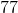Explanation:

To find the mean, we will use the following formula:Now, given the setWe can calculate the following: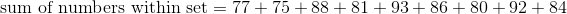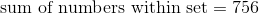We can also calculate the following: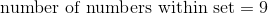because if we count, we can see there are 9 numbers in the set.

So, we can substitute. We get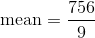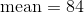Therefore, the mean score is 84.

### Example Question #63 : Statistics

Determine the mean of the numbers: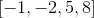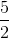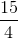Explanation:

The mean is the average of all the numbers in the data set.

Add all the numbers and divide the total by four.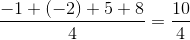Reduce the fraction.

The mean is:### Example Question #64 : Statistics

Determine the mean of the numbers: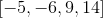Explanation:

The mean is the average of all the numbers in the set of numbers.

Add the numbers and divide the quantity by four.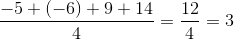The mean is:### All GED Math Resources